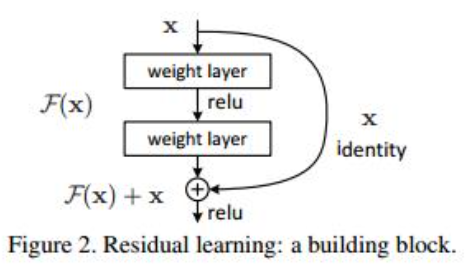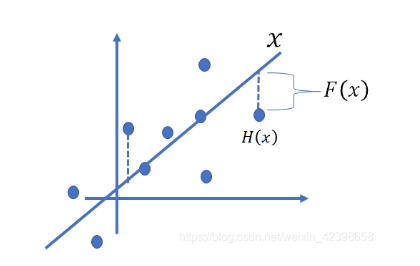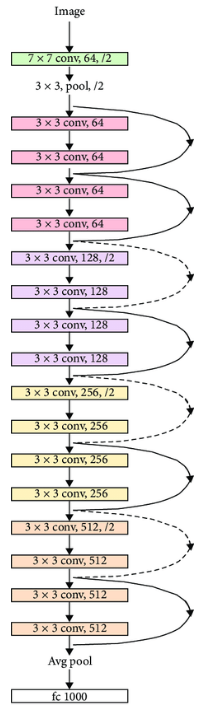# 深度学习——手动实现残差网络 辛普森一家人物识别

## 数据## ResNet介绍

1. 梯度消失：当使用深层的网络时（例如> 100）反向传播时会产生梯度消。由于参数初始化一般更靠近0，这样在训练的过程中更新浅层网络的参数时，很容易随着网络的深入而导致梯度消失，浅层的参数无法更新。

2. 退化问题：层数越多，训练错误率与测试错误率反而升高。举个例子，假设已经有了一个最优化的网络结构，是18层。当我们设计网络结构的时候，我们并不知道具体多少层次的网络时最优化的网络结构，假设设计了34层网络结构。那么多出来的16层其实是冗余的，我们希望训练网络的过程中，模型能够自己训练这16层为恒等映射，也就是经过这层时的输入与输出完全一样，这样子最终的结果和18层是一致的最优的。但是往往模型很难将这16层恒等映射的参数学习正确，那么就一定会不比最优化的18层网络结构性能好，所以随着网络深度增加，模型会产生退化现象。它不是由过拟合产生的，而是由冗余的网络层学习了不是恒等映射的参数造成的。

ResNet使用了一个新的思想，ResNet的思想是假设我们的网络，存在最优化的网络层次，那么往往我们设计的深层次网络是有很多网络层为冗余层的。那么我们希望这些冗余层能够完成恒等映射，保证经过该恒等层的输入和输出完全相同。ResNet就是在网络中添加shortcut，来构成一个个的残差块，从而解决梯度爆炸和网络退化。

## ResNet18网络结构## 项目思路

ResNet18是一个卷积神经网络。它的架构有18层。它在图像分类中是非常有用和有效的。首先是一个卷积层，内核大小为3x3，步幅为1。

## Data Transform:

``````    data = torchvision.datasets.ImageFolder(root=student.dataset,
transform=student.transform('train'))
batch_size=1, shuffle=True)
mean = torch.zeros(3)
std = torch.zeros(3)
images, labels = batch
for d in range(3):
mean[d] += images[:, d, :, :].mean()
std[d] += images[:, d, :, :].std()
mean.div_(len(data))
std.div_(len(data))
print(list(mean.numpy()), list(std.numpy()))
``````

## 超参数及其他设定

### Epochs, batch_size, learning rate:

`epochs = 120`, 如果太小，收敛可能不会结束。
`batch_size = 256`, 如果batch_size太小，可能会导致收敛速度过慢或损失不会减少
`lr = 0.001`，当学习率设置过大时，梯度可能会围绕最小值振荡，甚至无法收敛

### Loss function:

`torch.nn.CrossEntropyLoss() `是很适合图像作业的

## 代码

``````def transform(mode):
"""
//pytorch.org/vision/stable/transforms.html
You may specify different transforms for training and testing
"""

if mode == 'train':
return  transforms.Compose([
# transforms.Grayscale(num_output_channels=1),
transforms.RandomHorizontalFlip(),
transforms.RandomVerticalFlip(),
transforms.ToTensor(),
transforms.Normalize([0.42988312, 0.42988312, 0.42988312],
[0.17416202, 0.17416202, 0.17416202])
])
elif mode == 'test':
return  transforms.Compose([
# transforms.Grayscale(num_output_channels=1),
transforms.RandomHorizontalFlip(),
transforms.RandomVerticalFlip(),
transforms.ToTensor(),
transforms.Normalize([0.42988312, 0.42988312, 0.42988312],
[0.17416202, 0.17416202, 0.17416202])
])

``````

ResNet18手工搭建

``````class Network(nn.Module):
def __init__(self, num_classes=14):
super().__init__()
self.inchannel = 64
self.conv1 = nn.Sequential(
nn.Conv2d(3, 64, kernel_size=3, stride=1, padding=1, bias=False),
nn.BatchNorm2d(64),
nn.ReLU()
# nn.MaxPool2d(3, 1, 1)
)
self.layer1 = self.make_layer(ResBlock, 64, 2, stride=1)
self.layer2 = self.make_layer(ResBlock, 128, 2, stride=2)
self.layer3 = self.make_layer(ResBlock, 256, 2, stride=2)
self.layer4 = self.make_layer(ResBlock, 512, 2, stride=2)
self.fc = nn.Linear(512, num_classes)

def make_layer(self, block, channels, num_blocks, stride):
layers = []
for i in range(num_blocks):
if i == 0:
layers.append(block(self.inchannel, channels, stride))
else:
layers.append(block(channels, channels, 1))
self.inchannel = channels
return nn.Sequential(*layers)

def forward(self, x):
out = self.conv1(x)
out = self.layer1(out)
out = self.layer2(out)
out = self.layer3(out)
out = self.layer4(out)
out = self.avgpool(out)
out = out.reshape(out.size(0), -1)
out = self.fc(out)
return out

class ResBlock(nn.Module):
def __init__(self, inchannel, outchannel, stride=1):
super(ResBlock, self).__init__()
# two 3*3 kenerl size conv layers
self.left = nn.Sequential(
nn.Conv2d(inchannel, outchannel, kernel_size=3, stride=stride, padding=1, bias=False),
nn.BatchNorm2d(outchannel),
nn.ReLU(inplace=True),
nn.Conv2d(outchannel, outchannel, kernel_size=3, stride=1, padding=1, bias=False),
nn.BatchNorm2d(outchannel)
)
self.shortcut = nn.Sequential()
if stride != 1 or inchannel != outchannel:
# shortcut，1*1 kenerl size
# shortcut，这里为了跟2个卷积层的结果结构一致，要做处理
self.shortcut = nn.Sequential(
nn.Conv2d(inchannel, outchannel, kernel_size=1, stride=stride, bias=False),
nn.BatchNorm2d(outchannel)
)

def forward(self, x):
out = self.left(x)
# 将2个卷积层的输出跟处理过的x相加，实现ResNet的基本结构
out = out + self.shortcut(x)
out = F."externalu(out)
return out

``````